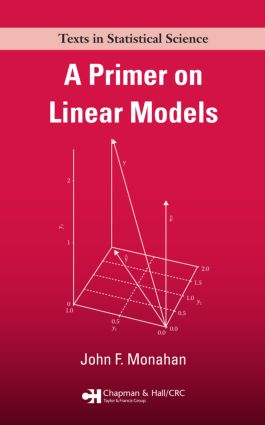# A Primer on Linear Models

## 1st Edition

Chapman and Hall/CRC

304 pages | 6 B/W Illus.

##### Purchasing Options:\$ = USD
Paperback: 9781420062014
pub: 2008-03-31
SAVE ~\$12.99
\$64.95
\$51.96
x
Hardback: 9781138469532
pub: 2017-08-09
SAVE ~\$41.00
\$205.00
\$164.00
x
eBook (VitalSource) : 9780429196065
pub: 2008-03-31
from \$31.48

FREE Standard Shipping!

### Description

A Primer on Linear Models presents a unified, thorough, and rigorous development of the theory behind the statistical methodology of regression and analysis of variance (ANOVA). It seamlessly incorporates these concepts using non-full-rank design matrices and emphasizes the exact, finite sample theory supporting common statistical methods.

With coverage steadily progressing in complexity, the text first provides examples of the general linear model, including multiple regression models, one-way ANOVA, mixed-effects models, and time series models. It then introduces the basic algebra and geometry of the linear least squares problem, before delving into estimability and the Gauss–Markov model. After presenting the statistical tools of hypothesis tests and confidence intervals, the author analyzes mixed models, such as two-way mixed ANOVA, and the multivariate linear model. The appendices review linear algebra fundamentals and results as well as Lagrange multipliers.

This book enables complete comprehension of the material by taking a general, unifying approach to the theory, fundamentals, and exact results of linear models.

### Reviews

"… I found the book very helpful. … the result is very nice, very readable, and in particular I like the idea of avoiding leaps in the development and proofs, or referring to other sources for the details of the proofs. This is a useful well-written instructive book."

International Statistical Review

"This work provides a brief, and also complete, foundation for the theory of basic linear models . . . can be used for graduate courses on linear models."

– Nicoleta Breaz, Zentralblatt Math

". . . well written . . . would serve well as the textbook for an introductory course in linear models, or as references for researchers who would like to review the theory of linear models."

Justine Shults, Department of Biostatistics, University of Pennsylvania School of Medicine, Journal of Biopharmaceutical Statistics

Preface

Examples of the General Linear Model

Introduction

One-Sample Problem

Simple Linear Regression

Multiple Regression

One-Way ANOVA

First Discussion

The Two-Way Nested Model

Two-Way Crossed Model

Analysis of Covariance

Autoregression

Discussion

The Linear Least Squares Problem

The Normal Equations

The Geometry of Least Squares

Reparameterization

Gram–Schmidt Orthonormalization

Estimability and Least Squares Estimators

Assumptions for the Linear Mean Model

Confounding, Identifiability, and Estimability

Estimability and Least Squares Estimators

First Example: One-Way ANOVA

Second Example: Two-Way Crossed without Interaction

Two-Way Crossed with Interaction

Reparameterization Revisited

Imposing Conditions for a Unique Solution to the Normal Equations

Constrained Parameter Space

Gauss–Markov Model

Model Assumptions

The Gauss–Markov Theorem

Variance Estimation

Implications of Model Selection

The Aitken Model and Generalized Least Squares

Application: Aggregation Bias

Best Estimation in a Constrained Parameter Space

Addendum: Variance of Variance Estimator

Distributional Theory

Introduction

Multivariate Normal Distribution

Chi-Square and Related Distributions

Distribution of Quadratic Forms

Cochran’s Theorem

Regression Models with Joint Normality

Statistical Inference

Introduction

Results from Statistical Theory

Testing the General Linear Hypothesis

The Likelihood Ratio Test and Change in SSE

First Principles Test and LRT

Confidence Intervals and Multiple Comparisons

Identifiability

Further Topics in Testing

Introduction

Reparameterization

Applying Cochran’s Theorem for Sequential SS

Orthogonal Polynomials and Contrasts

Pure Error and the Lack-of-Fit Test

Heresy: Testing Nontestable Hypotheses

Variance Components and Mixed Models

Introduction

Variance Components: One Way

Variance Components: Two-Way Mixed ANOVA

Variance Components: General Case

The Split Plot

Predictions and BLUPs

The Multivariate Linear Model

Introduction

The Multivariate Gauss–Markov Model

Inference under Normality Assumptions

Testing

Repeated Measures

Confidence Intervals

Appendix A: Review of Linear Algebra

Notation and Fundamentals

Rank, Column Space, and Nullspace

Some Useful Results

Solving Equations and Generalized Inverses

Projections and Idempotent Matrices

Trace, Determinants, and Eigenproblems

Definiteness and Factorizations

Appendix B: Lagrange Multipliers

Main Results

Bibliography

A Summary, Notes, and Exercises appear at the end of most chapters.

### Subject Categories

##### BISAC Subject Codes/Headings:
MAT029000
MATHEMATICS / Probability & Statistics / General